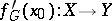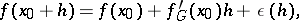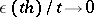# Gâteaux derivative

(diff) ← Older revision | Latest revision (diff) | Newer revision → (diff)
The derivative of a functional or a mapping which — together with the Fréchet derivative (the strong derivative) — is most frequently used in infinite-dimensional analysis. The Gâteaux derivative at a pointof a mappingfrom a linear topological spaceinto a linear topological spaceis the continuous linear mappingthat satisfies the conditionwhereasin the topology of(see also Gâteaux variation). If the mappinghas a Gâteaux derivative at the point, it is called Gâteaux differentiable. The theorem on differentiation of a composite function is usually invalid for the Gâteaux derivative. See also Differentiation of a mapping.